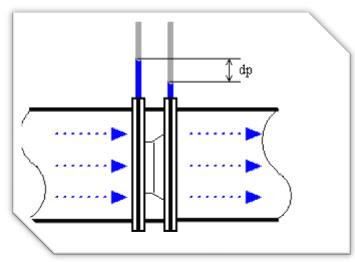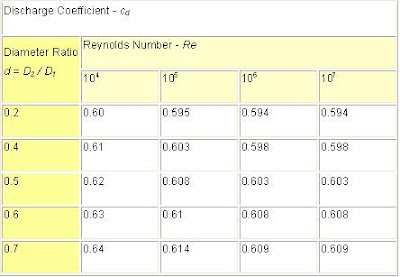# Orifice Meter

The orifice, nozzle and venturi flow rate meters use the Bernoulli Equation to calculate the fluid flow rate using pressure difference through obstructions in the flow.In a flow metering device based on the Bernoulli Equation the downstream pressure after an obstruction will be lower than the upstream pressure before. To understand orifice, nozzle and venturi meters it's therefore necessary to explore the Bernoulli Equation.

### THE BERNOULLI’S EQUATION - AN EXPLORATION

Assuming a horizontal flow (neglecting minor elevation differences between measuring points) the Bernoulli Equation can be modified to:
p1 + 1/2 ρ v12 = p2 + 1/2 ρ v22         (1)
p = pressure
ρ = density
v = flow velocity
The equation can be adapted to vertical flow by adding elevation heights h1 and h2.
Assuming uniform velocity profiles in the upstream and downstream flow - the Continuity Equation can be expressed as
q = v1 A1 = v2 A2         (2)
where
q = flow rate
A = flow area
Combining (1) and (2), assuming A2 < A1, gives the "ideal" equation:
q = A2 [ 2(p1 - p2) / ρ(1 - (A2 / A1)2) ]1/2         (3)
For a given geometry (A), the flow rate can be determined by measuring the pressure difference p1 - p2.
The theoretical flow rate q will in practice be smaller (2 - 40%) due to geometrical conditions.
The ideal equation (3) can be modified with a discharge coefficient:
q = cd A2 [ 2(p1 - p2) / ρ(1 - (A2 / A1)2) ]1/2         (3b)
where
cd = discharge coefficient
The discharge coefficient cd is a function of the jet size - or orifice opening - the
area ratio = Avc / A2
where
Avc = area in "vena contracta"
"Vena Contracta" is the minimum jet area that appears just downstream of the restriction. The viscous effect is usually expressed in terms of the nondimensional parameter Reynolds Number - Re.
Due to the Benoulli and Continuity Equation the velocity of the fluid will be at it's highest and the pressure at the lowest in "Vena Contracta". After the metering device the velocity will decrease to the same level as before the obstruction. The pressure recover to a pressure level lower than the pressure before the obstruction and adds a head loss to the flow.
Equation (3) can be modified with diameters to:
q = cd π/4 D22 [ 2(p1 - p2) / ρ(1 - d4) ]1/2         (4)
where
D2 = orifice, venturi or nozzle inside diameter
D1 = upstream and downstream pipe diameter
d = D2 / D1 diameter ratio
π = 3.14
Equation (4) can be modified to mass flow for fluids by simply multiplying with the density:
m = cd π/4 D22 ρ [ 2(p1 - p2) / ρ(1 - d4) ]1/2         (5)
When measuring the mass flow in gases, its necessary to considerate the pressure reduction and change in density of the fluid. The formula above can be used with limitations for applications with relatively small changes in pressure and density.Orifice Meter
ORIFICE METER
The orifice meter consists of a flat orifice plate with a circular hole drilled in it. There is a pressure tap upstream from the orifice plate and another just downstream. There are in general three methods of placing the taps. The coefficient of the meter depends upon the position of taps.
• Flange location - Tap location 1 inch upstream and 1 inch downstream from face of orifice
• "Vena Contracta" location - Tap location 1 pipe diameter (actual inside) upstream and 0.3 to 0.8 pipe diameter downstream from face of orifice
• Pipe location - Tap location 2.5 times nominal pipe diameter upstream and 8 times nominal pipe diameter downstream from face of orifice
The discharge coefficient - cd - varies considerably with changes in area ratio and the Reynolds number. A discharge coefficient cd = 0.60 may be taken as standard, but the value varies noticeably at low values of the Reynolds number.Coefficient of Discharge vs Reynold's number
The pressure recovery is limited for an orifice plate and the permanent pressure loss depends primarily on the area ratio. For an area ratio of 0.5, the head loss is about 70 - 75% of the orifice differential.
• The orifice meter is recommended for clean and dirty liquids and some slurry services.
• The rangeability is 4 to 1
• The pressure loss is medium
• Typical accuracy is 2 to 4% of full scale
• The required upstream diameter is 10 to 30
• The viscosity effect is high and the relative cost is low